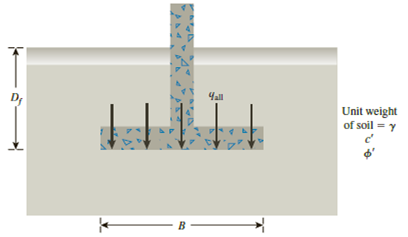Chapter 16, Problem 16.2PPrinciples of Geotechnical Enginee...

9th Edition
Braja M. Das + 1 other
ISBN: 9781305970939

Solutions

Chapter
SectionPrinciples of Geotechnical Enginee...

9th Edition
Braja M. Das + 1 other
ISBN: 9781305970939
Textbook Problem

Refer to Problem 16.1. If a square footing with dimension 2 m × 2 m is used instead of the wall footing, what would be the allowable bearing capacity?16.1 A continuous footing is shown in Figure 16.17. Using Terzaghi’s bearing capacity factors, determine the gross allowable load per unit area (qall) that the footing can carry. Assume general shear failure. Given: γ = 19 kN/m3, c′ = 31kN/m2, ϕ ′ = 28 ° , Df = 1.5 m, B = 2 m, and factor of safety = 3.5.Figure 16.17

To determine

Find the bearing capacity of square footing.

Explanation

Given information:

The unit weight of the soil γ is 19kN/m3.

The value of cohesion c is 31kN/m2.

The soil friction angle ϕ is 28°.

The depth of foundation Df is 1.5 m.

The width of footing B is 2 m.

The factor of safety Fs is 3.5.

The size of the square footing is (2m×2m).

Calculation:

Determine the ultimate bearing capacity of the soil (qu) using the relation.

qu=1.3cNc+qNq+0.4γBNγ=1.3cNc+γDfNq+0.4γBNγ (1)

Here, Nc is the contribution of cohesion, Nq is the contribution of surcharge, and Nγ is the contribution of unit weight of soil.

Refer Table 16.1, “Terzaghi’s bearing-capacity factors–Nc, Nq, and Nγ” in the textbook.

Take Nc as 31.61, Nq as 17

Still sussing out bartleby?

Check out a sample textbook solution.

See a sample solution

The Solution to Your Study Problems

Bartleby provides explanations to thousands of textbook problems written by our experts, many with advanced degrees!

Get Started

Find more solutions based on key concepts